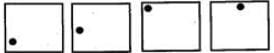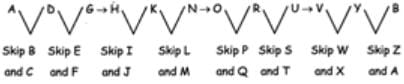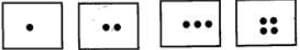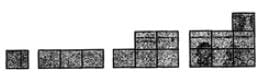# Test: Shapes

## 10 Questions MCQ Test Mathematics for Class 3 | Test: Shapes

Description
Attempt Test: Shapes | 10 questions in 15 minutes | Mock test for Class 3 preparation | Free important questions MCQ to study Mathematics for Class 3 for Class 3 Exam | Download free PDF with solutions
QUESTION: 1

### Which figure is different from the rest?

Solution:

Figure A is a square divided in 4 parts. Figure B is a circle divided in 2 parts. Figure C is a pentagon divided in 3 parts. Figure b is a hexagon divided in 6 parts. Hence, Figures A. B and D have even number of parts, but Figure C has odd number of parts and hence C is different from the rest.

QUESTION: 2

Solution:
QUESTION: 3

### What are the next two terms in the pattern?Solution:

The point is moving clockwise at a distance equal to half the length of side from the point in the previous term.

QUESTION: 4

What comes next in the sequence?

Solution:

Within each term each letter skips 2 alphabets to reach next; the next term starts with the letter following the last alphabet in the term before.QUESTION: 5

What comes next in the pattern?Solution:

The 1st term has 1 dot inside the rectangle. The 2nd term has 2 (1 + 1) dots inside the rectangle. The 3rd term has 3(2 + 1) dots inside the rectangle. The 4th term has 4(3 + 1) dots inside the rectangle. The 5th term has 5(4 + 1) dots inside the rectangle.

QUESTION: 6

What comes next in the pattern?
32, 30, 28, 26...........

Solution:

The difference between two terms is 2 in decrease order.
32 - 2 = 30
30 - 2 = 28
28 - 2 = 26
26 - 2 = 24

QUESTION: 7

What is rule of the pattern below?
2, 5, 11, 23, 47.........

Solution:

(2 x 2) + 1 = 5
(5 x 2) + 1 = 11
(11 x 2) + 1 = 23
(23 x 2) + 1 = 47

QUESTION: 8

What are the missing numbers in the pattern?
34,  ___,  42, 46, ___, 54

Solution:

The rule of the pattern is to add 4
34 + 4 = 38
38 + 4 = 42
42 + 4 = 46
46 + 4 = 50
50 + 4 = 54

QUESTION: 9

What comes next in the pattern?Solution:

The first term has 1 square. The 2nd term has 3 (1 + 2) squares. The 3rd term has 5 (3 + 2) squares. The 4th term has 7 (5 + 2) squares. The 5th term will have 9 (7 + 2) squares.

QUESTION: 10

Sahil started counting from 12. He is skip counting by 4. He made the following statements. A: 24 is the 2nd number in the list. B: 29 is a number in the list. C: 60 is the 13th number in the list. D: 35 is not a number in the list. Which option is correct?

Solution:

The list of the number will be 12, 16, 20, 24, 28, 32, 36, 40, 44, 48, 52, 56, 60, 64........Use Code STAYHOME200 and get INR 200 additional OFF Use Coupon Code